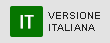# Antonio CAUSA

Researcher of Geometry [MAT/03]
Office: Dipartimento di Matematica e Informatica, ufficio 332
Email: causa@dmi.unict.it
Phone: 095 7383067
Fax: ++39 095 330094
antonio.causa5Antonio Causa was born in 1968, graduated in Physics at University of Catania in 1992 and he is a researcher in Geometry  at University of Catania since 1997.
He has taught courses of Geometry, Linear Algebra, Coding Theory at Faculty of Engineering, Mathematics, Informatics of the University of Catania since 1998.
His research interests mainly concern Algebraic Geometry, Coding Theory, Variational Inequalities.

1. Causa A., Jadamba B., Raciti F.  Decis. Econ. Finance Volume 40, pp. 159-175 (2017)  “A migration equilibrium model with uncertain data and movement costs”

2. Causa A, Raciti F. J. Optim. Theory Appl. Volume 156, pp. 524–528 (2013)  “A purely geometric approach to the problem of computing the projection of a point on a simplex”

3. Causa A. , R. Re  Annali di Matematica pure ed applicata, vol. 190, pagg. 55-59, (2011)  “On the maximum rank of a real binary form” doi: 10.1007/s10231-010-0137-2

4. Causa A., Raciti F.  Optimization Letters, volume 5, n. 3 pagg. 99-112 (2011) “Some remarks on the Walras equilibrium problem in Lebesgue spaces”, ISSN: 1862-4472 doi: 10.1007/s11590-010-0193-y

5. Arena G., Caruso A., Causa A. Rev. Mat. Iberoamericana Volume 26, Number 1, pp. 239-259 (2010) “Taylor formula on step two Carnot groups”

6. Causa A., Raciti F.  Journal of Optimization Theory and Applications, volume 145 n. 2, pagg. 235-248 (2010) “Lipschitz Continuity results for a Class of Variational Inequalities and Applications: a Geometric Approach” doi: 10.1007/s10957-009-9622-4

7. Causa A., Raciti F.  COMMUNICATIONS TO SIMAI CONGRESS, vol. 3, p. 229-241, (2009) “On the Modelling of the Time Dependent Walras Equilibrium Problem”ISSN: 1827-9015, doi: 10.1685/csc09229

8. Causa A., Re R.   Communications in Algebra, volume 36, Issue 3, pagg.      1041-1058, (2008) “Homogeneous nilpotent matrices in two variables” ISSN: 0092-7872, doi: 10.1080/00927870701776839

9. Arena G., Caruso A., Causa A  Le Matematiche Volume 60, pp. 375-383 (2005)  “Formula di Taylor nei Gruppi di Carnot ed applicazioni”

10. Causa A.  Le Matematiche  Volume 53  no. 1, pp. 155–175(1998),  “Sulle curve di genere Massimo”.

Teachings held in other departments

My research interests mainly concern Algebraic Geometry, Coding Theory, Variational Inequalities.
Some results have been obtained on the "Waring problem" for real binary symmetric tensors.
The Waring problem for symmetric tensors deals with the following problem: given a degree n polynomial in m variables find the (Waring) rank of f, i.e. the minimum number of summands that achieve the following equality: f=a1l1n +...+ aklkn with ai real coefficients and li linear forms in m variables.
In the context of Variational Inequalities it has been studied the regularity of the solution of some classes of variational inequalities.
Let K be a nonempty, closed and convex subset of the n-dimensional Euclidean space Rn, F : U → Rn a continuous mapping. The variational inequality problem (VI) is the problem of finding a point x* in K such that
F(x*)(y − x*) ≥ 0 ∀y in K.
If the set K and the mappings F are dependent by a (usually real) parameter t one can ask which properties solution x*(t) fulfills.
When the convex set K(t) is a polytope, i.e. a bounded intersection of a finite set of half-spaces, it has been studied Lipschitz continuity of solution x*(t).
During the last decades  many scholars have been studying the connections between commutative algebra and combinatorics. It has been noted that problems in the former can be translated in  the language of the latter and vice versa.
As a starting point some combinatorial objects as graphs and hypergraphs can be used to construct particular monomial ideals called Edge ideals and Covering ideals. In this context we are interested in studying some algebraic invariants such Betti numbers, Hilbert function, Castelnuovo-Mumford regularity, Waldschmidt constant of Edge ideals and Covering ideals associated to matroids or Steiner systems.
A Matroid is a hypergraph which consists of a a finite set M of elements together with a family of subsets of M, called independent sets, such that the empty set is independent, every subset of an independent set is independent, for every subset A of M, all maximum independent sets contained in A have the same number of elements.
Another interesting problem is the Ideal containment problem for Edge ideals and Covering ideals, i.e. decide for  which m and r there is the containment I(m)  is a subset of Ir, where I(m) is the symbolic power of the ideal I and Ir is the regular power of I.